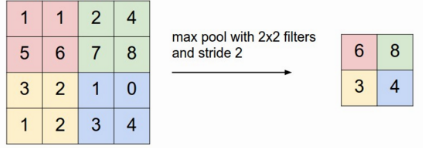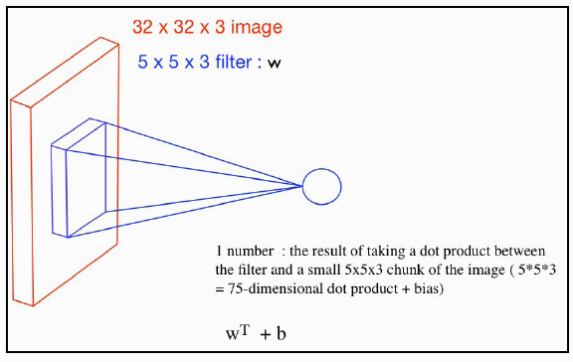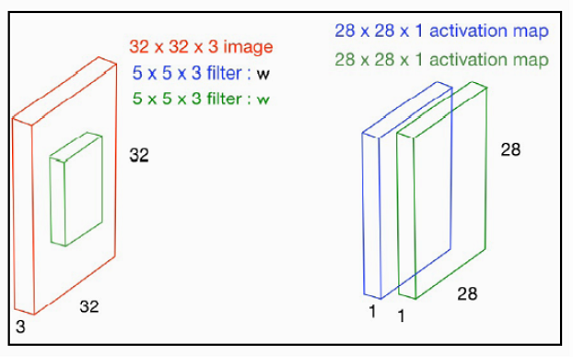## 2019/1/1

### Convolutional Neural Network (CNN) 原理

23 Convolutional Neural Network (CNN) 原理

🔶 Convolution operation (摺積操作)
Convolutional Neural Network (摺積神經網路 ) 指的是在神經網路中至少有一層的神經元使用摺積 (Convolution)操作。Convolution 的中文翻譯除摺積外，還有迴旋、卷積等，但我認為還是用摺積好。在電機領域裡的「訊號與系統」中，摺積是指將一個訊號輸入到一個系統的操作，假設訊號是$s(t)$，系統的脈衝響應是$h(t)$，所得的輸出訊號$y(t)$就是$s(t)$ 和 $h(t)$的摺積，數學表示是
$$y(t)=\int_{\tau=- \infty }^{\infty }s(\tau -t)h(\tau)d\tau$$ $$\: \: \: \: \: =\int_{\tau=-\infty }^{\infty }s(\tau )h(\tau-t)d\tau$$ 其中可見摺積的操作主要是先將兩函數之任一個反摺，之後再求積分，也因而叫摺積。

🔶 CNN 的特性
CNN在深度學習裡常用於影像辨識和自然語言處理(Natural language processing)，我們先來討影像辨識的問題。先來看看CNN有那些特性使其能成功地應用於影像辨識？

►階層式特徵攫取

► Local invariance and compositionality
Local invariance (位置無關) 是指關注的特徵不管出現在圖像的任何位置均可被偵測出來，亦即將圖片旋轉某角度後對偵測的結果還是不變，此特性在物體辨識的應用中相當重要，因我們事先並不知某特徵會出現在圖片的那一位置。在每一層中均有很多並列的kernels，可以想像每kernel 負責偵測一種特徵，所以不管特徵出現在任何位置均會被某一kernel 偵測出來，也因要實現此一特性，CNN中每一摺積層才會有很多 kernels 並列。 Compositionality 是指複合性，也就是先前函數的輸出是下一級函數的輸入，數學上可表示成如$f(g(h(x)))$的函數操作，也因有此一特性才能實現階層式特徵攫取。

► 權重係數共用和濾波器學習

► Local receptive field (區域接受場)

► 作動圖 (activation map)

🔶 CNN 各層和實例
► Convolutional layer (摺積層)

► Pooling Layer (池化層)池化的例子

► Dropout Layer (捨棄層)
Dropout layer 是用於隨機捨棄輸入tensor 的某些元素，最主要的功能是用來防範過適應。

► Fully-connected (FC) (全連接層)

► flat layer (展平層)

► CNN 實例CNN的實例

🔶 摺積操作輸出的 feature map 尺度與數目二維摺積單一kernel 對RGB 三色樣本兩個kernel 作用到彩色樣本圖